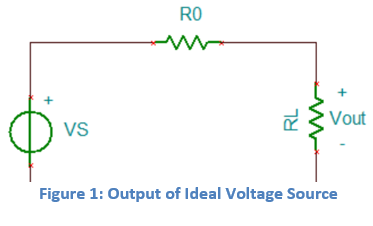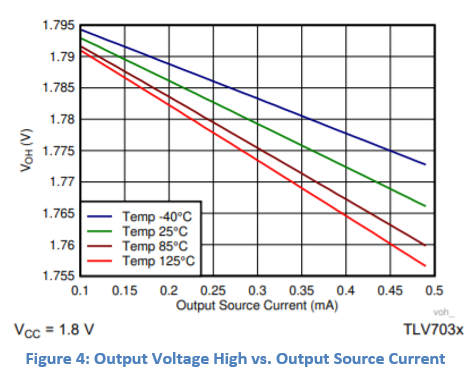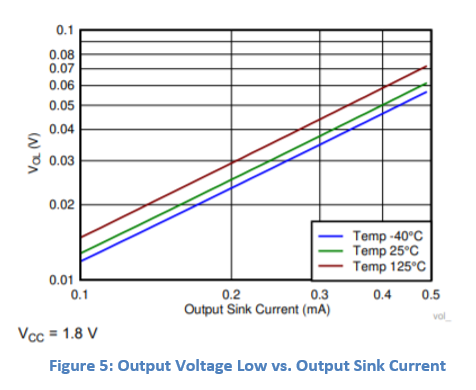If you have a related question, please click the "Ask a related question" button in the top right corner. The newly created question will be automatically linked to this question.

# [FAQ] How to determine if my comparator has a rail-to-rail output (RRO)?

Why is a rail-to-rail output (RRO) important, and how can I determine if my comparator has a RRO?

• Rail-to-rail output can be a desirable quality in a comparator, allowing hardware designers to take advantage of the comparator’s full voltage range. This is especially useful in low voltage applications where the switching window is already quite small. Many devices that operate on a battery, especially personal electronics, require accuracy when the supply voltage is low, as there is a lower margin on the logic threshold levels. For example, assuming a VIL max of 20% of 1.8V (0.36V) and VIH to be 80% of 1.8V (1.44V), it becomes critical to minimize any drop on the outputs, as the switching window has already been reduced to 1.08V total.

Most comparators have rail-to-rail output capability, but the specific behavior will depend on the loading at the output, as this will determine output proximity to the rail. With a light load (the impedance of the device connected at the comparator output is high), the comparator’s output voltage will be close to ideal, with output voltages swinging from the low supply voltage to the high supply voltage. In cases where the impedance of the device connected to the output is low, the opposite is true, and only a partial voltage swing may occur. Put simply, achieving rail-to-rail functionality on the comparator output depends directly on the system in which it is operating.To understand this better, let’s consider an ideal voltage source. The output of an ideal voltage source maintains its exact voltage value at Vout as long as it stays ideal (Ro is 0 ohms or RL is infinite). However, as we know, all real-world voltage sources have some small internal resistance, and all loads have some impedance that is less than infinite. As a result, the output voltage is never exactly the source voltage, since some small voltage drop must occur across the internal resistance.

In much the same way, the output of a comparator can be compared to the output of a voltage source. In an ideal world, the comparator’s output is always rail-to-rail. However, once a load RL becomes added to that output it may no longer be truly “rail-to-rail.” In both open-drain and push-pull devices, the output now sinks some current that lowers the output voltage. The degree to which the output voltage changes depends on the load via Ohm’s Law V=IR. An extremely large load RL will have a very large voltage drop, and will be “rail-to-rail” in practice, even though it may only reach 99% of the source rail. Decreasing the size of this load will decrease its voltage drop, taking the output of the comparator further away from the rail. In a push-pull scenario,

increasing the load size should also bring the output across that load closer to the rail. An extremely large load RL coupled with a very small internal resistance R0 could bring the output to within 99% of the rail value, which in practice, can be considered “rail-to-rail.”

This is exemplified by the output behavior of TLV703x/4x comparator family. In figures 4 and 5 below, the output varies based on changes in the resistance value, with the graph sloperepresenting the resistance. With an output high of 1.8V in figure 4, the graph shows a
decrease in output voltage as the resistance decreases, meaning that the output voltage becomes pulled further from the rail. Similarly, figure 5 shows how increasing the output voltage depends on increasing the output resistance. By increasing the resistance of the load, the comparator output draws closer to the rail.

In short, a comparator can practically function as rail-to-rail out given the proper load conditions.